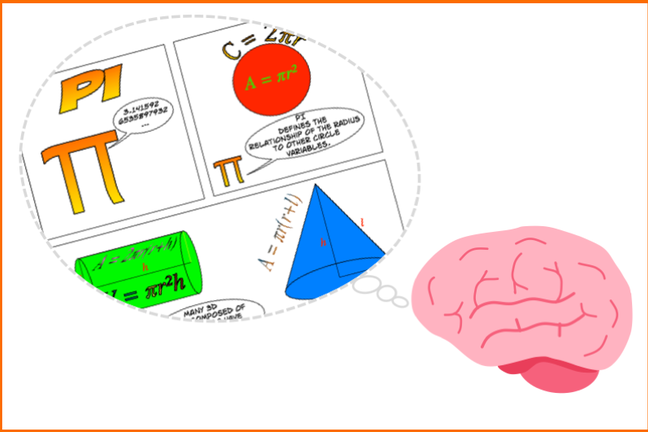## Want to keep learning?

This content is taken from the University of York's online course, The Biology of Bugs, Brains, and Beasts. Join the course to learn more.
2.11

## University of York# Activity: Learning Pi by association

Can you memorise the digits of $$\pi$$ (Pi)?

The mathematical constant $$\pi$$ (Pi) represents the ratio of a circle’s circumference to its diameter and we encounter it in various equations that we learn at school (such as area of a circle = $$\pi r^2$$). Pi is an irrational number (having infinite digits), but fortunately there is a button that easily inserts its value into your calculator. However, knowing the digits of Pi has fascinated mathematicians ever since Archimedes wrote an equation to calculate Pi. More recently, memorising the digits of Pi has become an obsession for some. The subject of learning Pi is called ‘piphilology’, and the record stands at over 70,000 digits!

In this activity you will devise strategies to help you memorise the value of Pi, using the fact that learning occurs by context and association. This is how mnemonics work. You could for example choose to make a humorous rhyme (a ‘piem’, where the number of letters in each word represents a digit from Pi), or learn by associating with historic memorable dates, or break it down into phone number-length chunks.

Post your ideas on Padlet and tell us how many digits of Pi you have been able to memorise using your ideas.

Here are the first 100 digits of Pi:

3.1415926535897932384626433832795028841971693993751058209749445923078164062862089986280348253421170679